Select Page

# Number fraction (num.fr.)

Num.fr.

### Definition in words

The number of component B divided by number of system 1

### Definition by equation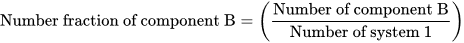### Systematic terms

Number fraction of component B in system 1

### Result type

Ratio scale
• Value sets are positive numerical values
• Algebraic operations are allowed (a=nb; a≠nb)
Numerical values from 0-∞

### Unit

10-18, 10-15, 10-12, 10-9, 10-6, 10-3, 1 (one), etc.

Unit, one (1) is omitted in the NPU syntax

### Reference

Ferard G, Dybkaer R, Fuentes-Arderiu X. Compendium of Terminology and Nomenclature of Properties in Clinical Laboratory Sciences : Recommendations 2016. 1 ed: Royal Society of Chemistry; 2016. 182 p.

### Allergology

Example 1
Generic abbreviated
NPU58094 Basocs(B)—Bee venom activated basophilocyte; num.fr.(i1; proc.) = ?
Full result NPU58094 Basocs(Blood)—Bee venom activated basophilocyte; number fraction(i1; procedure) = 0.34 x one
Abbreviated result NPU58094 Basocs(B)—Bee venom activated basophilocyte; num.fr.(i1; proc.) = 0.34
Written expression The number fraction of Bee venom activated basophilocyte in Mr. Smith’s Blood is [0.34]. The measurement is performed according to a procedure defined be the laboratory. Bee venom is defined by the preparation by the Clinical & Laboratory Standards Institute (CLSI, http://ila37.clsi.org/) current code, i3.
Equation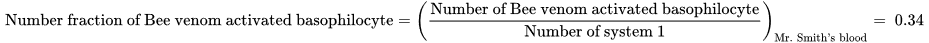### Clinical Biochemistry

Example 2
Generic abbreviated
NPU10756 Lkcs(Asc)—Neutrophilocytes; num.fr. = ?
Full result NPU10756 Leukocytes(Ascites)—Neutrophilocytes; number fraction = 0.75 x one
Abbreviated result NPU10756 Lkcs(Asc)—Neutrophilocytes; num.fr. = 0.75
Written expression The number fraction of Neutrophilocytes of all Leukocytes in Mrs. Smith’s Ascites is [0.75] .
Equation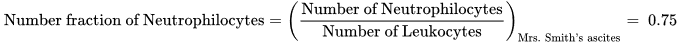### Clinical Immunology and Biobanking

Example 3
Generic abbreviated
NPU21798 Lymcs(B)—NK-lymphocytes; num.fr. = ?
Full result NPU21798 Lymphocytes(Blood)—NK-lymphocytes; number fraction = 0.04 x one
Abbreviated result NPU21798 Lymcs(B)—NK-lymphocytes; num.fr. = 0.04
Written expression The number fraction of NK-lymphocytes of all Lymphocytes in Mrs. Smith’s Blood is [0.04].
Equation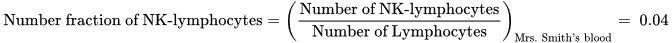### Clinical Microbiology

Example 4
Generic abbreviated
NPU16084 Ercs(B)—Erythrocytes(Plasmodium); num.fr. = ?
Full result NPU16084 Erythrocytes(Blood)—Erythrocytes(Plasmodium); number fraction = 0.15 x one
Abbreviated result NPU16084 Ercs(B)—Erythrocytes(Plasmodium); num.fr. = 0.15
Written expression The number fraction of Erythrocytes with Plasmodium of all Erythrocytes in Mr. Smith’s Blood is [0.15].
Equation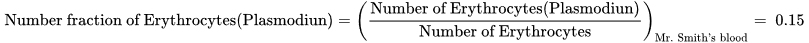### Molecular Biology and Genetics

Example 5
Generic abbreviated
NPU28669 IGKV3-20 gene(mRNA; spec.)—IGKV3-20 gene(hypermutation); num.fr.(proc.) = ?
Full result NPU28669 IGKV3-20 gene(mRNA; specification)—IGKV3-20 gene(hypermutation); number fraction(procedure) = 0.1 x one
Abbreviated result NPU28669 IGKV3-20 gene(mRNA; spec.)—IGKV3-20 gene(hypermutation); num.fr.(proc.) = 0.1
Written expression The number fraction of IGKV3-20 gene(hypermutation) of all mRNA IGKV3-20 gene in Mrs. Smith’s ovarian tumor is [0.1]. The measurement is performed according to a procedure defined be the laboratory.
Equation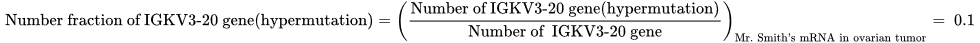Notes IThe system specification (IGKV3-20 gene(mRNA; specification)) has been stated as an ovarian tumor by the local laboratory.

### Reproduction and Fertility

Example 6
Generic abbreviated
NPU03459 Spmzoa—Spermatozoa(live); num.fr.(proc.) = ?
Full result NPU03459 Spermatozoa—Spermatozoa(live); number fraction(procedure) = 0.56 x one
Abbreviated result NPU03459 Spmzoa—Spermatozoa(live); num.fr.(proc.) = 0.56
Written expression The number fraction of alive Spermatozoa of all Mr. Smith’s Spermatozoa is [0.56]. The measurement is performed according to a procedure defined be the laboratory.
Equation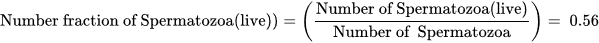### Thrombosis and Haemostasis

Example 7
Generic abbreviated
NPU26825 Trcs(B)—Thrombocytes(Aggregation, arachidonate-induced); num.fr.(proc.) = ?
Full result NPU26825 Thrombocytes(Blood)—Thrombocytes(Aggregation, arachidonate-induced); number fraction(procedure) = 0.27 x one
Abbreviated result NPU26825 Trcs(B)—Thrombocytes(Aggregation, arachidonate-induced); num.fr.(proc.) = 0.27
Written expression The number fraction of arachidonate-induced Thrombocytes(Aggregation) of all Thrombocytes in Mr. Smith’s Blood is [0.27]. The measurement is performed according to a procedure defined be the laboratory.
Equation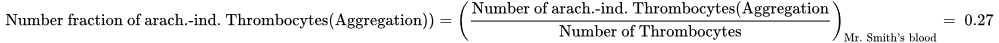### Reference

NPU database, https://www.ifcc.org/ifcc-scientific-division/sd-committees/c-npu/npusearch/ and http://www.labterm.dk/default.aspx
• # Kind-of-property

• ## Kind-of-quantity

### Reference

• Dybkaer R. Concept system on ‘quantity’: formation and terminology. Accredit Qual Assur. 2013;18(3):253-60.
• Dybkaer R. ISO terminological analysis of the VIM3 concepts ‘quantity’ and ‘kind-of-quantity’. Metrologia. 2010;47(3):127-34.
Date Term Note
1996-01-01 Number fraction Term established

QU50045